# Brother and sister

When I was 8, my sister was 4, now I am 18, how old is my sister?

Correct result:

s =  14

#### Solution:

$s=4+\left(18-8\right)=14$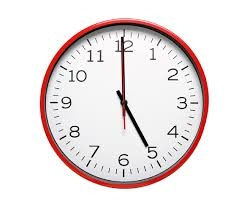We would be pleased if you find an error in the word problem, spelling mistakes, or inaccuracies and send it to us. Thank you!Tips to related online calculators
Do you have a linear equation or system of equations and looking for its solution? Or do you have quadratic equation?
Do you want to convert time units like minutes to seconds?

## Next similar math problems:Added together and write as decimal number: LXVII + MLXIV
• Roman numerals +Add up the number writtens in Roman numerals. Write the results as a decimal number.
• Roman numerals 2+Add up the number writtens in Roman numerals. Write the results as a roman numbers.
• Forest nurseryIn the forest nursery after winter, they found that 1/10 stems died out of them. For them, they land 193 new spruces. How many spruces are in the forest nursery?
• Six te 2If 3t-7=5t, then 6t=
• Simple equation 8Solve the following equation: 36=-(1+7x)-6(-7-x)
• If-then equationIf 5x - 17 = -x + 7, then x =
• Simple equation 1035= 7*3*x what is x?
• Eq1Solve equation: 4(a-3)=3(2a-5)
• Equation with one variableSolve the following equation with one unknown: 5(7s + 5) =130
• Minutes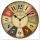Determine the difference in minutes: T1 = 2 3/20 h T2 = 2.3 h
• Flood waterFlood waters in some US village meant that the homes had to evacuate 364 people. 50 of them stayed at elementary schools, 59 them slept with their friends and others went to relatives. How many people have gone to relatives?
• Pupil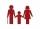I'm a primary school pupil. I attended the exercises of parents with children 1/4 of my age, 1/3 for drawing, and 1/6 for flute. For the first three years of my life, I had no ring, and I never went to two rings at the same time. How old am I?
• The rodThe rod has a length of 90cm. Half is painted blue, the third is red and the rest is yellow. How long is the yellow part of the rod?
• Father and son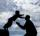Father is 44 years old, his son 16 years. Determine how many years ago was the father five times older than the son.
• I think numberI think number.When I add 841 to it and subtract 157, I get a number that is 22 greater than 996. What number I thinking?
• Drive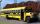At the bus stop 8 people take out and 10 people take into bus. Next stop 6 take out and 5 take in. On the third stop 6 take out and 3 take in. The bus traveled further with 39 people. How many passengers were originally at the bus?